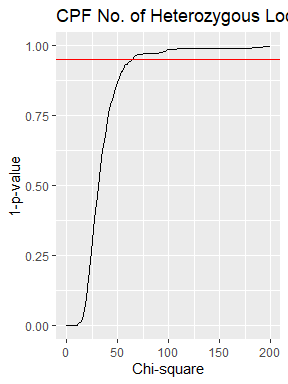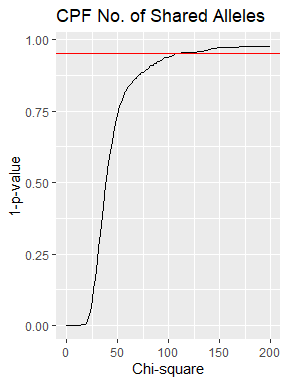Hypothesis Test

This vignette will introduce you to find the critical value for comparison of observed and expected obtained last step.

Simulate_DistK and Simulate_DistX simulate bundles of expected distributions for number of heterozygous loci and number of shared alleles respectively.

Simu_K <- Simulate_DistK(H,100,500)
Simu_X <- Simulate_DistX(e,100,500)

Dist_SimuChisq generates a bundle of chi-square values which can be distributed. ecdf build the cumulative probability functions for the chi-square values.

x2_K<-Dist_SimuChisq(Simu_K,ExpDist_K$Density,200) x2_X<-Dist_SimuChisq(Simu_X,ExpDist_X$Density,200)
P1<-ecdf(x2_K)
P2<-ecdf(x2_X)